# Complex number calculator

Rectangular form:
z = 25

Angle notation (phasor):
z = 25 ∠ 0°

Polar form:
z = 25 × (cos 0° + i sin 0°)

Exponential form:
z = 25 × ei 0

Polar coordinates:
r = |z| = 25 ... magnitude (modulus, absolute value)
θ = arg z = 0 rad = 0° = 0π rad ... angle (argument or phase)

Cartesian coordinates:
Cartesian form of imaginary number: z = 25
Real part: x = Re z = 25
Imaginary part: y = Im z = 0

### Calculation steps

1. Complex number: 3+4i
2. Complex number: 3-4i
3. Multiple: the result of step No. 1 * the result of step No. 2 = (3+4i) * (3-4i) = 3 * 3 + 3 * (-4i) + 4i * 3 + 4i * (-4i) = 9-12i+12i-16i2 = 9-12i+12i+16 = 9 + 16 +i(-12 + 12) = 25
alternative steps
5 × ei 0.2951672 × 5 × ei (-0.2951672) = 5 × 5 × ei (0.2951672+(-0.2951672)) = 25 × ei 0 =
This calculator does basic arithmetic on complex numbers and evaluates expressions in the set of complex numbers. As imaginary unit use i or j (in electrical engineering), which satisfies basic equation i2 = −1 or j2 = −1. The calculator also converts a complex number into angle notation (phasor notation), exponential, or polar coordinates (magnitude and angle). Enter expression with complex numbers like 5*(1+i)(-2-5i)^2

Complex numbers in the angle notation or phasor (polar coordinates r, θ) may you write as rLθ where r is magnitude/amplitude/radius, and θ is the angle (phase) in degrees, for example, 5L65 which is the same as 5*cis(65°).
Example of multiplication of two imaginary numbers in the angle/polar/phasor notation: 10L45 * 3L90.

Why the next complex numbers calculator when we have WolframAlpha? Because Wolfram tool is slow and some features such as step by step are charged premium service.
For use in education (for example, calculations of alternating currents at high school), you need a quick and precise complex number calculator.

## Basic operations with complex numbers

We hope that work with the complex number is quite easy because you can work with imaginary unit i as a variable. And use definition i2 = -1 to simplify complex expressions. Many operations are the same as operations with two-dimensional vectors.

### Addition

Very simple, add up the real parts (without i) and add up the imaginary parts (with i):
This is equal to use rule: (a+bi)+(c+di) = (a+c) + (b+d)i

(1+i) + (6-5i) = 7-4i
12 + 6-5i = 18-5i
(10-5i) + (-5+5i) = 5

### Subtraction

Again very simple, subtract the real parts and subtract the imaginary parts (with i):
This is equal to use rule: (a+bi)+(c+di) = (a-c) + (b-d)i

(1+i) - (3-5i) = -2+6i
-1/2 - (6-5i) = -6.5+5i
(10-5i) - (-5+5i) = 15-10i

### Multiplication

To multiply two complex numbers, use distributive law, avoid binomials, and apply i2 = -1.
This is equal to use rule: (a+bi)(c+di) = (ac-bd) + (ad+bc)i

(1+i) (3+5i) = 1*3+1*5i+i*3+i*5i = 3+5i+3i-5 = -2+8i
-1/2 * (6-5i) = -3+2.5i
(10-5i) * (-5+5i) = -25+75i

### Division

The division of two complex numbers can be accomplished by multiplying the numerator and denominator by the denominator's complex conjugate. This avoids imaginary unit i from the denominator. If the denominator is c+di, to make it without i (or make it real), multiply with conjugate c-di:

(c+di)(c-di) = c2+d2

(10-5i) / (1+i) = 2.5-7.5i
-3 / (2-i) = -1.2-0.6i
6i / (4+3i) = 0.72+0.96i

### Absolute value or modulus

The absolute value or modulus is the distance of the image of a complex number from the origin in the plane. The calculator uses the Pythagorean theorem to find this distance. Very simple, see examples: |3+4i| = 5
|1-i| = 1.4142136
|6i| = 6
abs(2+5i) = 5.3851648

### Square root

Square root of complex number (a+bi) is z, if z2 = (a+bi). Here ends simplicity. Because of the fundamental theorem of algebra, you will always have two different square roots for a given number. If you want to find out the possible values, the easiest way is probably to go with De Moivre's formula. Here our calculator is on edge, because square root is not a well defined function on complex number. We calculate all complex roots from any number - even in expressions:

sqrt(9i) = 2.1213203+2.1213203i
sqrt(10-6i) = 3.2910412-0.9115656i
pow(-32,1/5)/5 = -0.4
pow(1+2i,1/3)*sqrt(4) = 2.439233+0.9434225i
pow(-5i,1/8)*pow(8,1/3) = 2.3986959-0.4771303i

### Square, power, complex exponentiation

Our calculator can power any complex number to any integer (positive, negative), real, or even complex number. In other words, we calculate 'complex number to a complex power' or 'complex number raised to a power'...
Famous example:
${i}^{i}={e}^{-\pi \mathrm{/}2}$
i^2 = -1
i^61 = i
(6-2i)^6 = -22528-59904i
(6-i)^4.5 = 2486.1377428-2284.5557378i
(6-5i)^(-3+32i) = 2929449.03994-9022199.58262i
i^i = 0.2078795764
pow(1+i,3) = -2+2i

### Functions

sqrt
Square Root of a value or expression.
sin
the sine of a value or expression. Autodetect radians/degrees.
cos
the cosine of a value or expression. Autodetect radians/degrees.
tan
tangent of a value or expression. Autodetect radians/degrees.
exp
e (the Euler Constant) raised to the power of a value or expression
pow
Power one complex number to another integer/real/complex number
ln
The natural logarithm of a value or expression
log
The base-10 logarithm of a value or expression
abs or |1+i|
The absolute value of a value or expression
phase
Phase (angle) of a complex number
cis
is less known notation: cis(x) = cos(x)+ i sin(x); example: cis (pi/2) + 3 = 3+i
conj
conjugate of complex number - example: conj(4i+5) = 5-4i

#### Examples:

cube root: cuberoot(1-27i)
roots of Complex Numbers: pow(1+i,1/7)
phase, complex number angle: phase(1+i)
cis form complex numbers: 5*cis(45°)
The polar form of complex numbers: 10L60
complex conjugate calculator: conj(4+5i)
equation with complex numbers: (z+i/2 )/(1-i) = 4z+5i
system of equations with imaginary numbers: x-y = 4+6i; 3ix+7y=x+iy
De Moivre's theorem - equation: z^4=1
multiplication of three complex numbers: (1+3i)(3+4i)(−5+3i)
Find the product of 3-4i and its conjugate.: (3-4i)*conj(3-4i)
operations with complex numbers: (3-i)^3

## Complex numbers in word problems:

• ABS CNCalculate the absolute value of complex number -15-29i.
• The modulus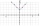Find the modulus of the complex number 2 + 5i
• Complex number coordinatesWhich coordinates show the location of -2+3i
• Mappings of complex numbers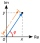Find the images of the following points under mappings: z=3-2j w=2zj+j-1
• Goniometric form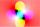Determine the goniometric form of a complex number z = √ 110 +4 i.
• Is complexAre these numbers 2i, 4i, 2i + 1, 8i, 2i + 3, 4 + 7i, 8i, 8i + 4, 5i, 6i, 3i complex?
• Linear imaginary equationGiven that 2(z+i)=i(z+i) "this is z star" Find the value of the complex number z.
• De Moivre's formula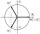There are two distinct complex numbers z, such that z3 is equal to 1 and z is not equal to 1. Calculate the sum of these two numbers.
• Im>0?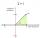Is -10i a positive number?
• Linear combination of complex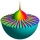If z1=5+3i and z2=4-2i, write the following in the form a+bi a) 4z1+6z2 b) z1*z2
• ReciprocalCalculate reciprocal of z=0.8-1.8i:
• Imaginary numbersFind two imaginary numbers whose sum is a real number. How are the two imaginary numbers related? What is its sum?
• Log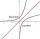Calculate value of expression log |3 +7i +5i2| .